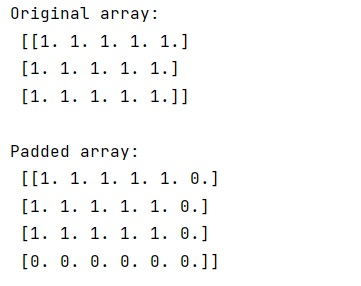# How to pad NumPy array with zeros?

Learn, how to pad NumPy array with zeros in Python?
Submitted by Pranit Sharma, on January 03, 2023

NumPy is an abbreviated form of Numerical Python. It is used for different types of scientific operations in python. Numpy is a vast library in python which is used for almost every kind of scientific or mathematical operation. It is itself an array which is a collection of various methods and functions for processing the arrays.

## Padding NumPy array with zeros

Padding of an array is the process of inserting some extra values either along the rows or columns.

We need to pad a 2D NumPy array with zeros using NumPy. Numpy provides us with a method called numpy.pad(). This method pads an array. It takes arguments like-array which has to be padded and pad_width which is the number of values padded to the edges of each axis. ((before_1, after_1), ... (before_N, after_N)) unique pad widths for each axis. (before, after) or ((before, after),) yields the same before and after pad for each axis. (pad,) or it is a shortcut for before = after = pad width for all axes.

Let us understand with the help of an example,

## Python code to pad NumPy array with zeros

```# Import numpy
import numpy as np

# Creating a numpy array
arr = np.array([[ 1.,  1.,  1.,  1.,  1.],[ 1.,  1.,  1.,  1.,  1.],[ 1.,  1.,  1.,  1.,  1.]])

# Display original array
print("Original array:\n",arr,"\n")

res = np.pad(arr, [(0, 1), (0, 1)], mode='constant')

# Display result
```

Output: